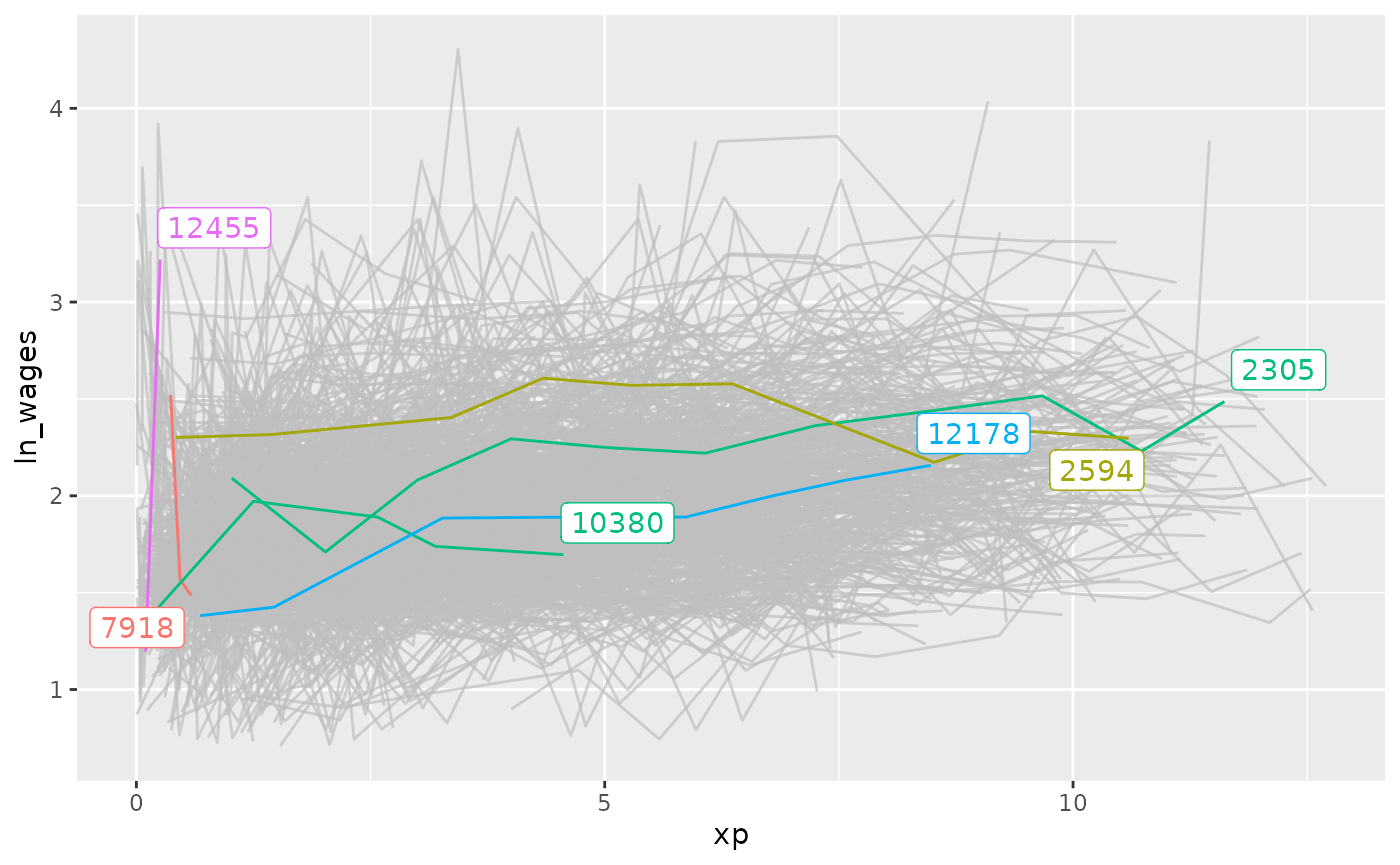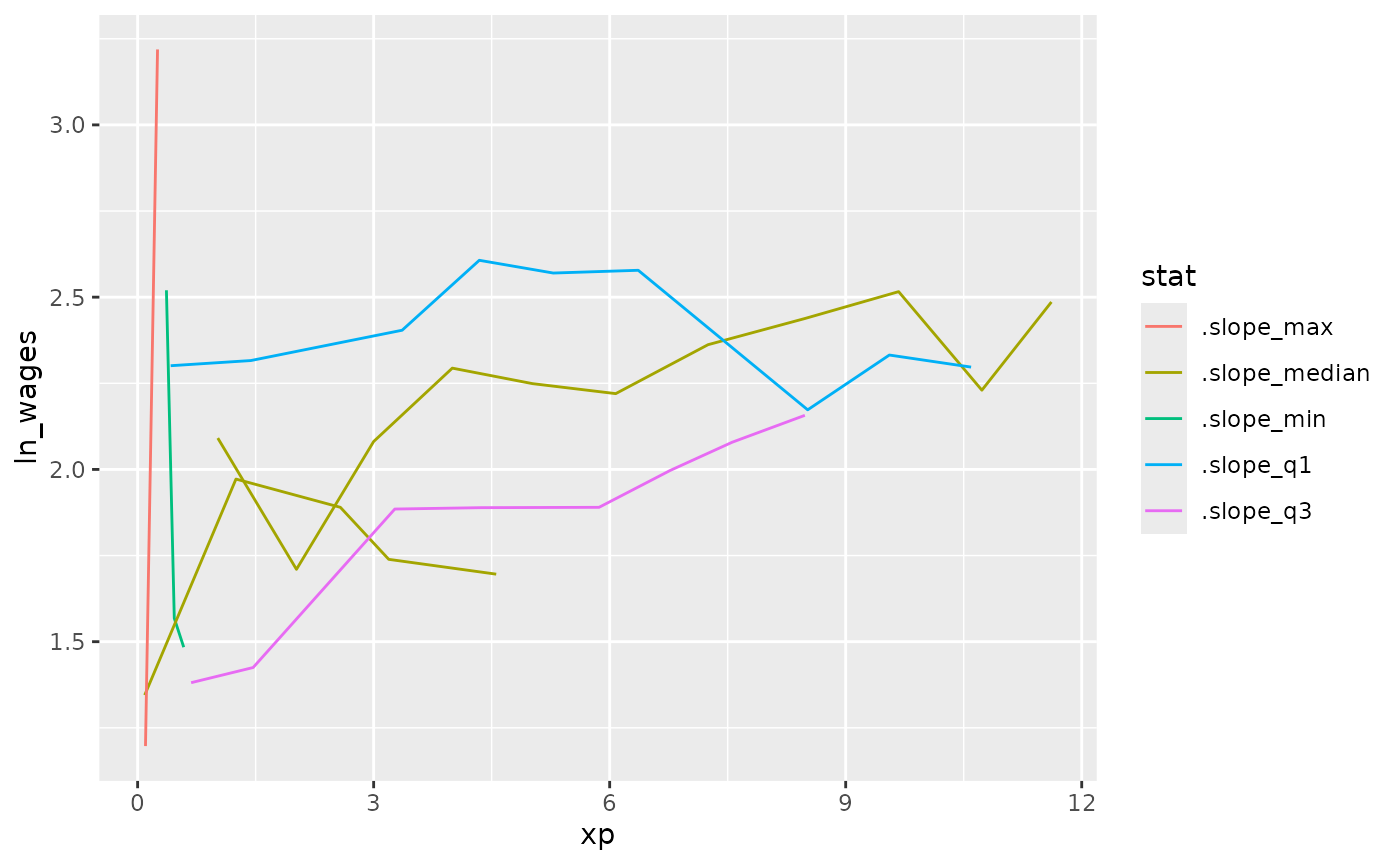This vignette unpacks how to find interesting individuals.

Let’s say we calculate a slope for each individual key, using keys_slope():

wages_slope <- key_slope(wages, ln_wages ~ xp)
wages_slope
#> # A tibble: 888 x 3
#>       id .intercept .slope_xp
#>    <int>      <dbl>     <dbl>
#>  1    31       1.41    0.101
#>  2    36       2.04    0.0588
#>  3    53       2.29   -0.358
#>  4   122       1.93    0.0374
#>  5   134       2.03    0.0831
#>  6   145       1.59    0.0469
#>  7   155       1.66    0.0867
#>  8   173       1.61    0.100
#>  9   206       1.73    0.180
#> 10   207       1.62    0.0884
#> # … with 878 more rows


This is neat! But now we want to know those keys that are nearest to some set of summary statistics of the slope. By nearest here we mean which values have the smallest numerical difference.

Let’s say the five number summary:

summary(wages_slope\$.slope_xp)
#>     Min.  1st Qu.   Median     Mean  3rd Qu.     Max.     NA's
#> -4.57692 -0.00764  0.04796  0.06235  0.09447 13.21569       38


If want to find those individuals that have slopes near these values.

We can do this using keys_near(), which returns those nearest to some summary statistics. In this case, it is the five number summary. In the next section we describe how you can provide your own named list of functions to use.


wages_slope_near <- wages_slope %>%
keys_near(key = id,
var = .slope_xp)

wages_slope_near
#> # A tibble: 6 x 5
#>      id .slope_xp stat  stat_value stat_diff
#>   <int>     <dbl> <fct>      <dbl>     <dbl>
#> 1  2305   0.0480  med      0.0480  0.0000498
#> 2  2594  -0.00768 q_25    -0.00769 0.0000127
#> 3  7918  -4.58    min     -4.58    0
#> 4 10380   0.0479  med      0.0480  0.0000498
#> 5 12178   0.0946  q_75     0.0947  0.0000579
#> 6 12455  13.2     max     13.2     0


We can then join this information back against the data and plot those interesting individuals:


wages_slope_near %>%
left_join(wages, by = "id") %>%
ggplot(aes(x = xp,
y = ln_wages,
group = id,
colour = stat)) +
geom_line()You could also, with a bit of work, show these lines against the background using gghighlight

library(gghighlight)
wages %>%
left_join(wages_slope_near, by = "id") %>%
as_tibble() %>%
ggplot(aes(x = xp,
y = ln_wages,
group = id,
colour = stat)) +
geom_line() +
gghighlight(!is.na(stat))
#> Warning: Tried to calculate with group_by(), but the calculation failed.
#> Falling back to ungrouped filter operation...
#> label_key: id# Specify your own summaries for keys_near

You can specify your own list of summaries to pass to keys_near. For example, you could create your own summaries to give a sense of range. Note that the functions here start with b_, and are b_summaries provided by brolgar that have sensible defaults. You can read about them here, or with ?b_summaries

l_ranges <- list(min = b_min,
range_diff = b_range_diff,
max = b_max,
iqr = b_iqr)

wages %>%
key_slope(formula = ln_wages ~ xp) %>%
keys_near(key = id,
var = .slope_xp,
funs = l_ranges)
#> # A tibble: 4 x 5
#>      id .slope_xp stat       stat_value stat_diff
#>   <int>     <dbl> <fct>           <dbl>     <dbl>
#> 1  7918    -4.58  min            -4.58   0
#> 2  9357     0.103 iqr             0.102  0.000139
#> 3 12455    13.2   range_diff     17.8    4.58
#> 4 12455    13.2   max            13.2    0


# Implementation of keys_near

If you are interested in the specifics of how keys_near() works, this section describes how it is implemented in brolgar.

To get the data into the right format, there are a few steps.

First, we need to get the data into a format where we have all the statistics that we are interested in, along with the id, and the statistic of interest.

We can fit a linear model for each key in the dataset using key_slope().

wages_slope <- key_slope(wages, ln_wages ~ xp)

wages_slope
#> # A tibble: 888 x 3
#>       id .intercept .slope_xp
#>    <int>      <dbl>     <dbl>
#>  1    31       1.41    0.101
#>  2    36       2.04    0.0588
#>  3    53       2.29   -0.358
#>  4   122       1.93    0.0374
#>  5   134       2.03    0.0831
#>  6   145       1.59    0.0469
#>  7   155       1.66    0.0867
#>  8   173       1.61    0.100
#>  9   206       1.73    0.180
#> 10   207       1.62    0.0884
#> # … with 878 more rows


We can then perform a summary of the statistic of interest, in this case the slope.

wages_slope_all_stats <- wages_slope %>%
mutate_at(.vars = vars(.slope_xp),
.funs = list(.slope_min = b_min,
.slope_max = b_max,
.slope_median = b_median,
.slope_q1 = b_q25,
.slope_q3 = b_q75)) %>%
select(id,
starts_with(".slope"))

wages_slope_all_stats
#> # A tibble: 888 x 7
#>       id .slope_xp .slope_min .slope_max .slope_median .slope_q1 .slope_q3
#>    <int>     <dbl>      <dbl>      <dbl>         <dbl>     <dbl>     <dbl>
#>  1    31    0.101       -4.58       13.2        0.0480  -0.00769    0.0947
#>  2    36    0.0588      -4.58       13.2        0.0480  -0.00769    0.0947
#>  3    53   -0.358       -4.58       13.2        0.0480  -0.00769    0.0947
#>  4   122    0.0374      -4.58       13.2        0.0480  -0.00769    0.0947
#>  5   134    0.0831      -4.58       13.2        0.0480  -0.00769    0.0947
#>  6   145    0.0469      -4.58       13.2        0.0480  -0.00769    0.0947
#>  7   155    0.0867      -4.58       13.2        0.0480  -0.00769    0.0947
#>  8   173    0.100       -4.58       13.2        0.0480  -0.00769    0.0947
#>  9   206    0.180       -4.58       13.2        0.0480  -0.00769    0.0947
#> 10   207    0.0884      -4.58       13.2        0.0480  -0.00769    0.0947
#> # … with 878 more rows


We then need to convert this into long format

wages_slope_all_stats_long <-
wages_slope_all_stats %>%
gather(key = "stat",
value = "stat_value",
-id,
-.slope_xp)

wages_slope_all_stats_long
#> # A tibble: 4,440 x 4
#>       id .slope_xp stat       stat_value
#>    <int>     <dbl> <chr>           <dbl>
#>  1    31    0.101  .slope_min      -4.58
#>  2    36    0.0588 .slope_min      -4.58
#>  3    53   -0.358  .slope_min      -4.58
#>  4   122    0.0374 .slope_min      -4.58
#>  5   134    0.0831 .slope_min      -4.58
#>  6   145    0.0469 .slope_min      -4.58
#>  7   155    0.0867 .slope_min      -4.58
#>  8   173    0.100  .slope_min      -4.58
#>  9   206    0.180  .slope_min      -4.58
#> 10   207    0.0884 .slope_min      -4.58
#> # … with 4,430 more rows


We can then calculate the difference between each stat and the slope, .slope_xp:

stats_diff <-
wages_slope_all_stats_long %>%
mutate(stat_diff = abs(.slope_xp - stat_value))

stats_diff
#> # A tibble: 4,440 x 5
#>       id .slope_xp stat       stat_value stat_diff
#>    <int>     <dbl> <chr>           <dbl>     <dbl>
#>  1    31    0.101  .slope_min      -4.58      4.68
#>  2    36    0.0588 .slope_min      -4.58      4.64
#>  3    53   -0.358  .slope_min      -4.58      4.22
#>  4   122    0.0374 .slope_min      -4.58      4.61
#>  5   134    0.0831 .slope_min      -4.58      4.66
#>  6   145    0.0469 .slope_min      -4.58      4.62
#>  7   155    0.0867 .slope_min      -4.58      4.66
#>  8   173    0.100  .slope_min      -4.58      4.68
#>  9   206    0.180  .slope_min      -4.58      4.76
#> 10   207    0.0884 .slope_min      -4.58      4.67
#> # … with 4,430 more rows


With stats diff, we can then group by the stat, and find return those rows with the smallest difference between the statistic and the value:

top_stats_diff <-
stats_diff %>%
group_by(stat) %>%
top_n(-1,
wt = stat_diff)

top_stats_diff
#> # A tibble: 6 x 5
#> # Groups:   stat 
#>      id .slope_xp stat          stat_value stat_diff
#>   <int>     <dbl> <chr>              <dbl>     <dbl>
#> 1  7918  -4.58    .slope_min      -4.58    0
#> 2 12455  13.2     .slope_max      13.2     0
#> 3  2305   0.0480  .slope_median    0.0480  0.0000498
#> 4 10380   0.0479  .slope_median    0.0480  0.0000498
#> 5  2594  -0.00768 .slope_q1       -0.00769 0.0000127
#> 6 12178   0.0946  .slope_q3        0.0947  0.0000579

top_stats_diff %>%
left_join(wages, by = "id") %>%
ggplot(aes(x = xp,
y = ln_wages,
group = id,
colour = stat)) +
geom_line()We can see that we get the same output using keys_near():

wages %>%
key_slope(ln_wages ~ xp) %>%
keys_near(key = id,
var = .slope_xp) %>%
left_join(wages, by = "id") %>%
ggplot(aes(x = xp,
y = ln_wages,
group = id,
colour = stat)) +
geom_line()